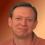## Wednesday, April 23, 2008

I love puzzles, brainteasers, logic riddles, and paradoxes.

Here's an interesting paradox. How many of the following five statements are false?

1. Three of these five statements are false.
2. 2 + 2 = 5
3. 3 +1 = 4
4. 4 x 2 = 8
5. 6 - 1 = 4

Statements 2 and 5 are clearly false. But what about statement 1? If it's true, there are three false statements. But where is the 3rd false statement? If it's false, then there are three false statements, which makes it true, which brings the number of false statement back to two. But, if there are only two false statements, then statement 1 is also false...

1.Weird. That's pretty cool.

2.Paradoxes are pretty easy to create then, aren't they?

3.You didn't answer the question! How many of those statements are false? Tell me now!

4.I can imagine someone seriously depleting their aspirin supply trying to answer that question!

5.So is saying Jesus was 100% man and 100% God a paradox or a contradiction?

6.7.All of those statements could be true or false if you change the numbering system.

For example, in base 4, 3+1 = 10

8.Good point, Perry. Though, according to the law of non-contradiction, two contradictory statements cannot both be true at the same time in the same sense.

9.Well, don't principles of quantum physics violate this Law of Non-Contradiction?

Light is both a particle and a wave but can't be.

A table is composed entirely of wood but simultaneously composed mostly of empty space.

10.Your statement about light is in the form of {P & Q}, since light exhibits the properties of both a particle and a wave, rather than {P & ~P}.

If you said, "Light is a particle and it is not the case that light is a particle" {P & ~P}, that would be a contradictory statement if applied at the same time and in the same sense.

In your table example, the two statements also do not contradict each other, since wood itself is composed mostly of empty space. So, the statement could be, "A is composed entirely of B. And B is composed of C & D," where C is empty space. No contradiction there.

Also, you can't deny laws of logic without using them to do so.